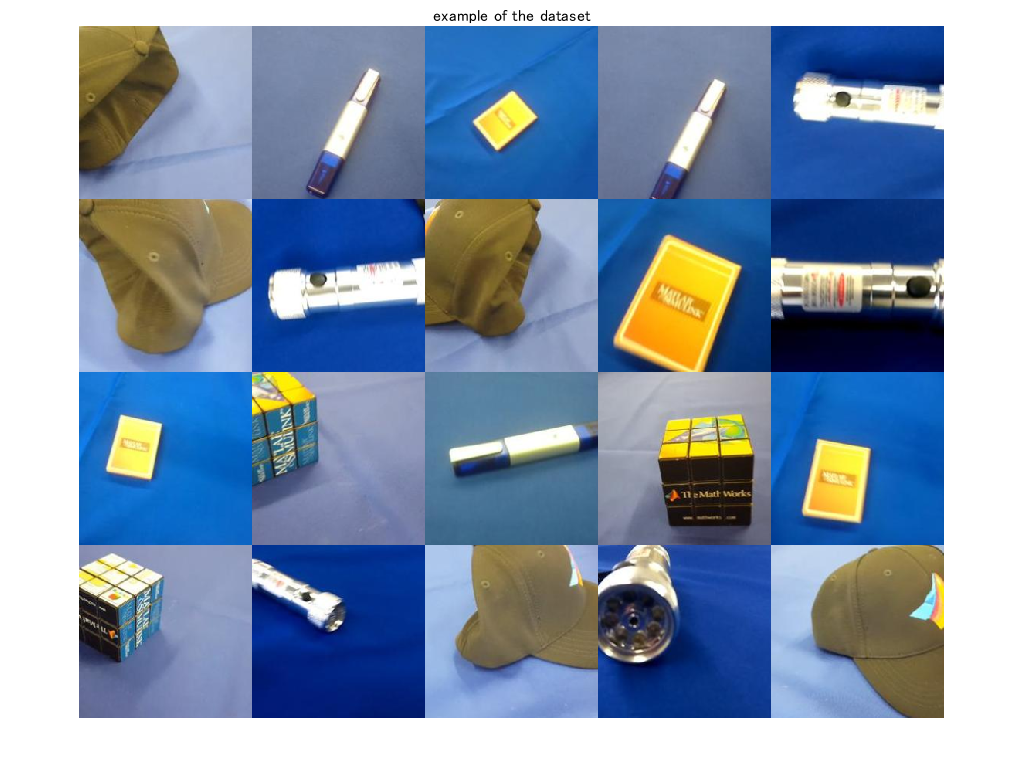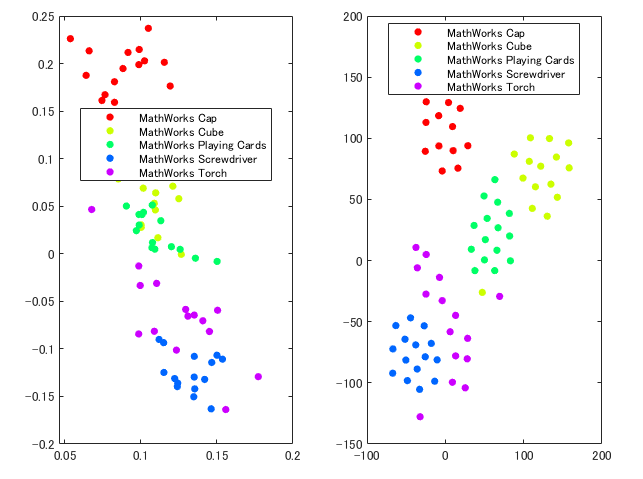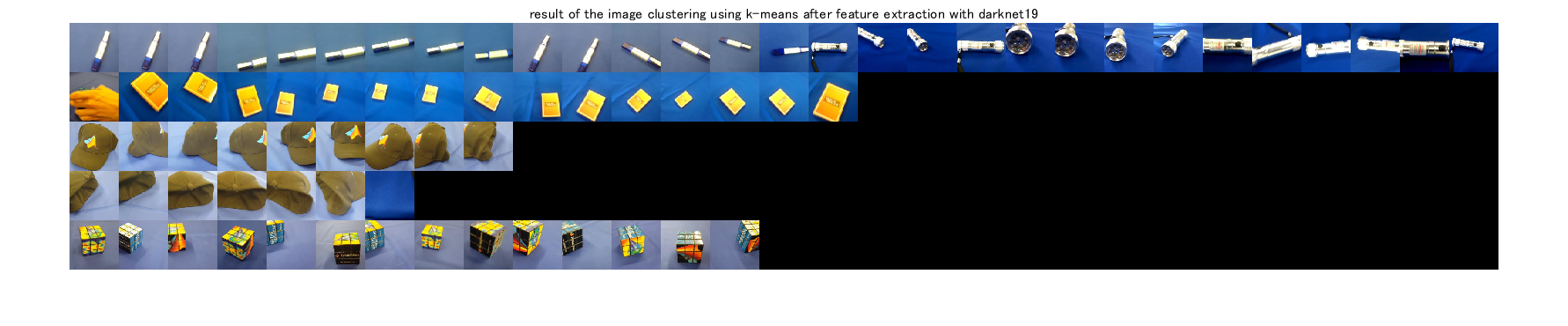## Image clustering and dimension reduction using CNN

version 1.2 (2.33 MB) by
This demo shows how to perform image clustering and dimension reduction using a pre-trained network. 学習済みネットワークを利用し、画像のクラスタリングや次元圧縮を行います。

Updated 25 Jul 2021

From GitHub# Dimension reduction and clustering for images

[English]

This demo shows how to perform image clustering and dimension reduction using a pre-trained network. The network has learned rich feature representations for a wide range of images. The feature extraction with the pre-trained network can be utilized for image clustering.

This demo did

2. feature extraction with the network

3. dimension reduction with the extracted features

4. image clustering with k-means algorithm

The illustrations in the thumbnail were obtained from https://www.irasutoya.com/

[Japanese]

このデモでは、学習済みの深層学習ネットワークやクラスタリングアルゴリズムを用いて、画像の教師なし分類を行います。MathWorksの`MerchData`を用いて、`resnet-18`による特徴抽出の後に、k-meansアルゴリズムにより分類を行います。

# Preparation

• We use a dataset called MearchData provided by Mathworks
• Load a pre-trained network called darknet19
• Load images from the dataset
``````clear;clc;close all
% unzip the zip file of MearchData
unzip('MerchData.zip');
``````

import a pre-trained network called `resnet18`

``````net=resnet18;
% load the images into the image data store called imds
imds = imageDatastore('MerchData','IncludeSubfolders',true,'LabelSource','foldernames');
% use augmented image datastore for image augmentation
augImds=augmentedImageDatastore(net.Layers(1, 1).InputSize(1:2),imds);
``````

Display some images from the dataset

``````% randomly extract image index to display some images
idx=randperm(numel(imds.Files),20);
% to show the tiled images
figure;montage(imgEx.input);title('example of the dataset')
``````Feature extraction using a pre-trained network

``````% Gather label information from the image datastore
Labels=imds.Labels;
% count the number of images
numClass=numel(countcats(Labels));
% feature extraction with the pre-trained network
feature=squeeze(activations(net,augImds,'fc1000'));
``````

# Dimension reduction with Principal component analysis and t-sne

``````figure;
% conduct a principal component analysis for the dimension reduction
A=pca(feature,"Centered",true);
subplot(1,2,1)
gscatter(A(:,1),A(:,2),Labels)
subplot(1,2,2)
% perform t-sne for the dimension reduction
T=tsne(feature');
gscatter(T(:,1),T(:,2),Labels)
``````# Image clustering using k-means after feature extraction with resnet-18

``````% perform k-means algorithm
% please note that as the result is dependent on the initial point in the algorithm, the
% result would not be same
C=kmeans(feature',numClass,"Start","plus");
% confirm the number of images in the largest group
[~,Frequency] = mode(C);
sz=net.Layers(1, 1).InputSize(1:2);
% prepare a matrix to show the clustering result
I=zeros(sz(1)*numClass,sz(2)*Frequency,3,'uint8');
% loop over the class to display images assigned to the group
for i=1:numClass
% read the images assigned to the group
% use the function "find" to find out the index of the i-th group image
% tile the images extracted above
I((i-1)*sz(1)+1:i*sz(1),1:sz(2)*numel(find(C==i)),:)=cat(2,ithGroup.input{:});
end
figure;imshow(I);title('result of the image clustering using k-means after feature extraction with darknet19')
``````### Cite As

Kenta (2022). Image clustering and dimension reduction using CNN (https://github.com/KentaItakura/Dimension-reduction-and-clustering-for-images-using-MATLAB/releases/tag/1.2), GitHub. Retrieved .

##### MATLAB Release Compatibility
Created with R2020a
Compatible with any release
##### Platform Compatibility
Windows macOS Linux